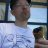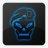# Plot Price every N minutes#### korygill

##### Active member
VIP
Getting the math right to plot price every N minutes is probably the hardest part about this indicator. The rest is pretty straightforward.

Here is an example with 12 hours (720 minutes) and 6 hours (360 minutes).Code looks like this:

Code:
``````#
# PlotTimeOfDay
#
# Author: Kory Gill, @korygill
# Paypal: paypal.me/korygill
#
declare upper;
declare once_per_bar;

input TimeOfDayEST = 930; # 24 hour time EST
input IntervalMinutes = 720; #hint IntervalMinutes: 12hr==720, 6hr==360
input Data = open;

def bn = BarNumber();
def nan = double.NaN;

def sft = SecondsFromTime(TimeOfDayEST);

def tt = if bn == 1
then nan
else if IsNaN(Data)
then tt
else if sft % (IntervalMinutes*60) == 0
then Data
else tt;

plot TimeTracker = tt;
TimeTracker.SetPaintingStrategy(PaintingStrategy.HORIZONTAL);``````
Link to the image/flex grid above

https://tos.mx/g8Btv1r

Kory Gill, @korygill

•T

##### Active member
2019 Donor
Getting the math right to plot price every N minutes is probably the hardest part about this indicator. The rest is pretty straightforward.

Here is an example with 12 hours (720 minutes) and 6 hours (360 minutes).Code looks like this:

Code:
``````#
# PlotTimeOfDay
#
# Author: Kory Gill, @korygill
# Paypal: paypal.me/korygill
#
declare upper;
declare once_per_bar;

input TimeOfDayEST = 930; # 24 hour time EST
input IntervalMinutes = 720; #hint IntervalMinutes: 12hr==720, 6hr==360
input Data = open;

def bn = BarNumber();
def nan = double.NaN;

def sft = SecondsFromTime(TimeOfDayEST);

def tt = if bn == 1
then nan
else if IsNaN(Data)
then tt
else if sft % (IntervalMinutes*60) == 0
then Data
else tt;

plot TimeTracker = tt;
TimeTracker.SetPaintingStrategy(PaintingStrategy.HORIZONTAL);``````
Link to the image/flex grid above

https://tos.mx/g8Btv1r

Kory Gill, @korygill
This is it thank
Getting the math right to plot price every N minutes is probably the hardest part about this indicator. The rest is pretty straightforward.

Here is an example with 12 hours (720 minutes) and 6 hours (360 minutes).Code looks like this:

Code:
``````#
# PlotTimeOfDay
#
# Author: Kory Gill, @korygill
# Paypal: paypal.me/korygill
#
declare upper;
declare once_per_bar;

input TimeOfDayEST = 930; # 24 hour time EST
input IntervalMinutes = 720; #hint IntervalMinutes: 12hr==720, 6hr==360
input Data = open;

def bn = BarNumber();
def nan = double.NaN;

def sft = SecondsFromTime(TimeOfDayEST);

def tt = if bn == 1
then nan
else if IsNaN(Data)
then tt
else if sft % (IntervalMinutes*60) == 0
then Data
else tt;

plot TimeTracker = tt;
TimeTracker.SetPaintingStrategy(PaintingStrategy.HORIZONTAL);``````
Link to the image/flex grid above

https://tos.mx/g8Btv1rPlot value of indicator over top of price? Questions 0Plot a box for average daily price movement? Questions 3